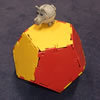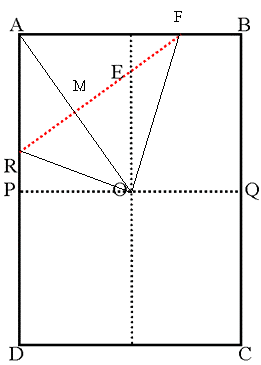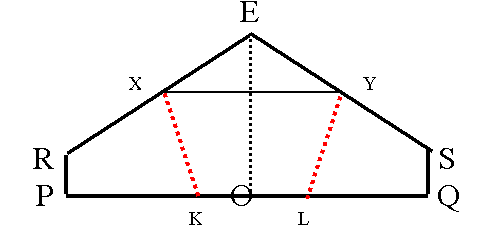#### You may also like### Proximity

We are given a regular icosahedron having three red vertices. Show that it has a vertex that has at least two red neighbours.### Platonic Planet

Glarsynost lives on a planet whose shape is that of a perfect regular dodecahedron. Can you describe the shortest journey she can make to ensure that she will see every part of the planet?### Three Cubes

Can you work out the dimensions of the three cubes?

# Dodecawhat

##### Age 14 to 16Challenge Level

Thank you Andrei from School No. 205, Bucharest, Romania for this solution.1

1. The angles of a regular pentagon must all be 108 °.
a) First I looked at the possibility of working with A4 paper.In the problem, I have to put A over O. So, triangles ARF and ORF are congruent, AM = MO and AO is perpendicular on FR.

As before, I note the smaller side of the paper (AB), with length x. Consequently, AD is $x\sqrt2$.

As P is on the middle of AD, AP = $x\sqrt2/2$. OP is x/2.

Triangle AOP is a right-angled triangle. As AP is parallel to EO, angles PAO and EOA are equal and, from the ratio of the side lengths of the paper, we get angle PAO = $tan^{-1}$ $(1\sqrt2)$ = 35.264 degrees.

As triangle MEO is also a right-angled triangle, angle EOM + angle MEO = $90^{\circ}$, so angle MEO is 54.736 degrees (to 3 decimal places).

But the figures (rectangle and then the pentagon) are symmetrical in respect to EO, so angle RES is two times angle MEO.

Angle RES = 2 $*$ MEO = $109.472^{\circ}$

The result is not $108^{\circ}$. The error is not so important, it is of the order of 1.4%

$${ {109.5^{\circ} - 108^{\circ}\over 108^{\circ}} = 1.4\%}$$

b) Now I have to calculate the new dimensions of the paper, so that I obtain a regular pentagon.

To make a regular pentagon in this way the ratio of the side lengths of the paper would have to be equal to tan 540 , that is in the ratio 1.376 to 1 (to 4 significant figures) rather than 1.414 to 1 as in A4 paper.In the figure angle RES has a measure of 108 °, XY is parallel to PQ and XK and YL are angle bisectors of angles RXY and SYX respectively.

Angles EXY and EYX are both equal to 36 °. Consequently angles RXY and XYS both have a measure of 144 °. Now, I calculate angle KXY:

KXY = RXY/2 = 72 °

Now, I calculate angles EXK and EYL (the angles of the pentagon):

EXK = EYL = 72 ° + 36 ° = 108 °

I observe that the other two remaining angles are also congruent (by symmetry), both having a measure of 108 °.

So, I proved that in this case I obtain a regular pentagon.

2 . The ratio of the lengths of A4 paper is $\sqrt2$ to $1$.
So, let the side of an A4 paper have lengths $x$ and $x\sqrt 2$.

Now, I write the lengths of the sides: $AB = x$ $AD =(x\sqrt{2})$ $AE = (x\sqrt{2/2})$
The ratio of sides EF to AE is: $EF/AE = x/ (x\sqrt 2/2)= 2/\sqrt2 = \sqrt2$

Continuing is the same manner, I observe that the smaller and the bigger A-paper all have the same proportions of length.